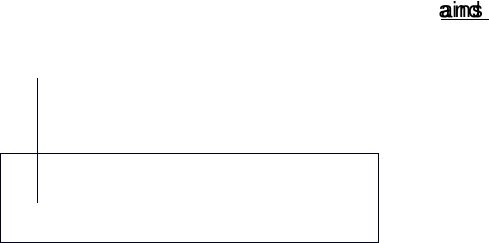Study Guides (400,000)
CA (150,000)
UTSG (10,000)
Geography (100)
Final

# Week 2 Study Notes

Department
Geography
Course Code
GGR270H1
Professor
Damian Dupuy
Study Guide
Final

Page:
of 4GGR 270 Lecture 2 September 22, 2010
Variables and Data
Variable
oCharacteristic of the population that changes or varies over time
oExamples include temperature, income, education, etc...
oObserve and measure variables
oTwo key categories
Quantitative (numerical)
Discrete or continuous
Qualitative (non-numerical)
Male/female, species
Data
oResults from measuring variables set of measurements
oDifferent categories: Univariate, Bivariate, Multivariate
Variables Scales of Measurement I
Scale defines amount of information a variable contains and what statistical
techniques can be used
Four scales:
Nominal
www.notesolution.com
oOrdinal
oInterval
oRatio
*Its easier to go from something complex into something simpler
Variables Scales of Measurement I I
Nominal
Lowest scale of measurement, no numerical value attached
Classifies observations into mutually exclusive and collectively exhaustive groups
Often called categorical data
Ex: occupation type, gender, place of birth
Ordinal
Stronger scale of measurement as it allows data to be ordered or ranked
Ex: 12 largest towns in a region, income by group (high, middle, low)
Interval
Unit distance separating numbers is important
Ex: Temperature (°C, F), taxable income (\$)
Ratio
www.notesolution.com
Strongest scale of measurement
Ratios of distance on a number scale
Presence of an absolute zero
Ex: Temperature (Kelvin), Income from all sources (\$), population in a city
*In practice we consider interval/ratio scales together
*in theory, 4 scales
*In practice, 3 scales
Describing Data I
Pie Charts: Circular graph where measurements are distributed among categories
Bar graphs: Graph where measurements are distributed among categories
Relative Frequency Histogram
Graphs quantitative, rather than qualitative data
Vertical axis (Y) shows how often measurements fall into a particular class or
subinterval
Classes are plotted on the horizontal (X) axis
Rules of thumb:
o5-12 intervals or categories
o1+3.3log
10 (# of observations)
oCategories must be mutually exclusive and collectively exhaustive
oIntervals should be the same width (Largest # - Smallest # / # of classes)
www.notesolution.com